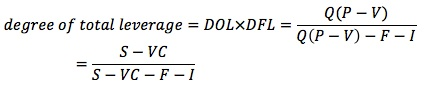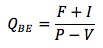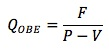### Why should I choose AnalystNotes?

Simply put: AnalystNotes offers the best value and the best product available to help you pass your exams.

##### Subject 3. Total Leverage and Breakeven Points
Operating leverage (first-stage leverage) affects EBIT, while financial leverage (second-stage leverage) affects earnings after interests and taxes (net income), which are the earnings available to shareholders. Financial leverage further magnifies the impact of operating leverage on earnings per share (EPS) due to changes in sales.

Both operating leverage and financial leverage contribute to the risk associated with a firm's future cash flows. The degree of total leverage (DTL) combines DOL and DFL, and measures the impact of a given percentage change in sales on EPS.If both DOL and DFL are high, a small change in sales leads to wide fluctuations in EPS.

The breakeven point is the volume of sales at which total costs equal total revenues, causing net income to equal zero: PQ - VQ - F - I = 0. The breakeven number of units, QBE, is:The operating breakeven point is the number of outputs at which revenues = operating costs: PQOBE = VQOBE + F. QOBE is:Consider a project where the fixed costs are \$10,000, the variable costs are \$2 per unit, the selling price per unit is \$4, and the interest expense is \$1,000. The breakeven sales quantity is 11,000 / (4 - 2) = 5,500 units and the operating breakeven sales quantity is 10,000 / (4 - 2) = 5,000 units.

In general, the farther unit sales are from the breakeven point for high-leverage companies, the greater the magnifying effect of this leverage.

Learning Outcome Statements

d. calculate the breakeven quantity of sales and determine the company's net income at various sales levels;

e. calculate and interpret the operating breakeven quantity of sales.

CFA® 2021 Level I Curriculum, , Volume 4, Reading 34

User Comment
farhan92 guys you can do this on the ba ii ..2nd 6 (breakeven) and fill in the deets!

for break even point you would have to make FC=10+1 (basically add the interest expense)
lordcomas Good one farhan92,
khalifa92 i do understand the concept behind it but why are excluding the variable cost
melmay11 Thank you Farhan92! Great tip!
MathLoser @khalifa92: breakeven, of course.